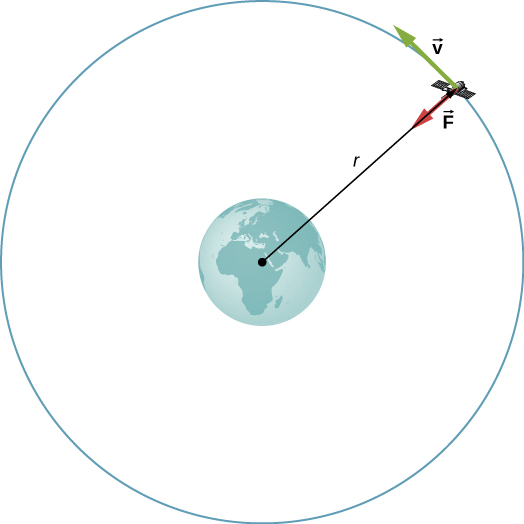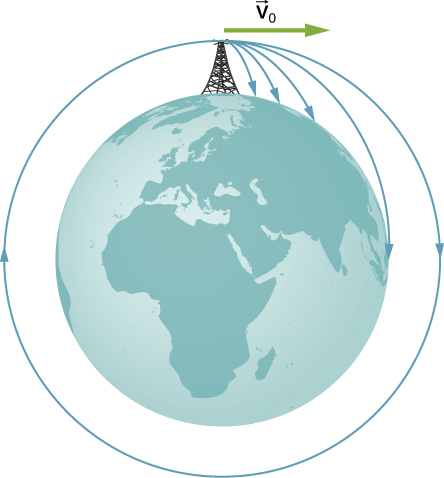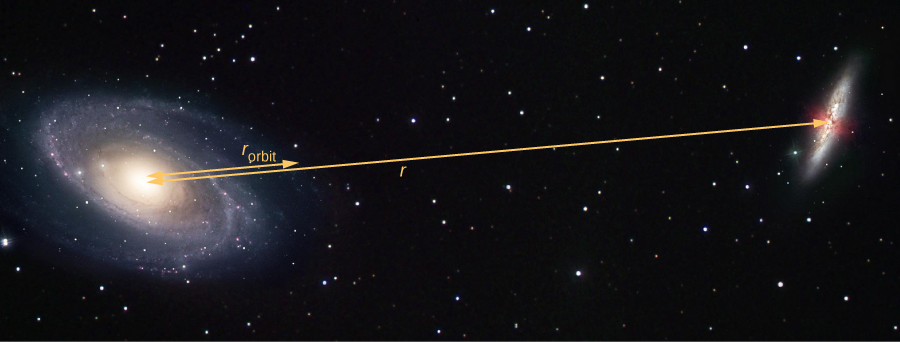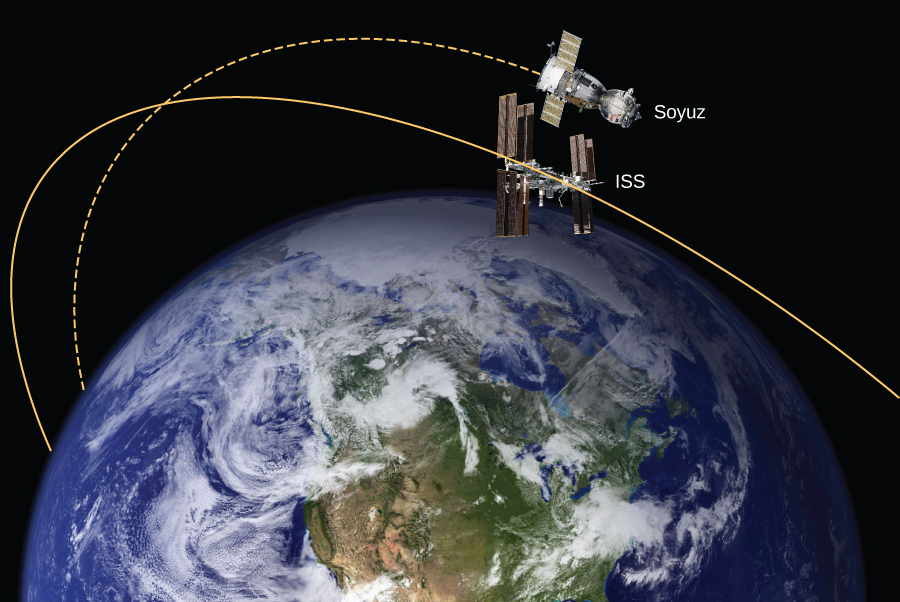Gravitation

# Satellite Orbits and Energy

### Learning Objectives

By the end of this section, you will be able to:

• Describe the mechanism for circular orbits
• Find the orbital periods and speeds of satellites
• Determine whether objects are gravitationally bound

The Moon orbits Earth. In turn, Earth and the other planets orbit the Sun. The space directly above our atmosphere is filled with artificial satellites in orbit. We examine the simplest of these orbits, the circular orbit, to understand the relationship between the speed and period of planets and satellites in relation to their positions and the bodies that they orbit.

### Circular Orbits

As noted at the beginning of this chapter, Nicolaus Copernicus first suggested that Earth and all other planets orbit the Sun in circles. He further noted that orbital periods increased with distance from the Sun. Later analysis by Kepler showed that these orbits are actually ellipses, but the orbits of most planets in the solar system are nearly circular. Earth’s orbital distance from the Sun varies a mere 2%. The exception is the eccentric orbit of Mercury, whose orbital distance varies nearly 40%.

Determining the orbital speed and orbital period of a satellite is much easier for circular orbits, so we make that assumption in the derivation that follows. As we described in the previous section, an object with negative total energy is gravitationally bound and therefore is in orbit. Our computation for the special case of circular orbits will confirm this. We focus on objects orbiting Earth, but our results can be generalized for other cases.

Consider a satellite of mass m in a circular orbit about Earth at distance r from the center of Earth ((Figure)). It has centripetal acceleration directed toward the center of Earth. Earth’s gravity is the only force acting, so Newton’s second law gives

$\frac{Gm{M}_{\text{E}}}{{r}^{2}}=m{a}_{\text{c}}=\frac{m{v}_{\text{orbit}}^{2}}{r}.$
A satellite of mass m orbiting at radius r from the center of Earth. The gravitational force supplies the centripetal acceleration.We solve for the speed of the orbit, noting that m cancels, to get the orbital speed

${v}_{\text{orbit}}=\sqrt{\frac{G{M}_{\text{E}}}{r}}.$

Consistent with what we saw in (Figure) and (Figure), m does not appear in (Figure). The value of g, the escape velocity, and orbital velocity depend only upon the distance from the center of the planet, and not upon the mass of the object being acted upon. Notice the similarity in the equations for ${v}_{\text{orbit}}$ and ${v}_{\text{esc}}$. The escape velocity is exactly $\sqrt{2}$ times greater, about 40%, than the orbital velocity. This comparison was noted in (Figure), and it is true for a satellite at any radius.

To find the period of a circular orbit, we note that the satellite travels the circumference of the orbit $2\pi r$ in one period T. Using the definition of speed, we have ${v}_{\text{orbit}}=2\pi r\text{/}T$. We substitute this into (Figure) and rearrange to get

$T=2\pi \sqrt{\frac{{r}^{3}}{G{M}_{\text{E}}}}.$

We see in the next section that this represents Kepler’s third law for the case of circular orbits. It also confirms Copernicus’s observation that the period of a planet increases with increasing distance from the Sun. We need only replace ${M}_{\text{E}}$ with ${M}_{\text{Sun}}$ in (Figure).

We conclude this section by returning to our earlier discussion about astronauts in orbit appearing to be weightless, as if they were free-falling towards Earth. In fact, they are in free fall. Consider the trajectories shown in (Figure). (This figure is based on a drawing by Newton in his Principia and also appeared earlier in Motion in Two and Three Dimensions.) All the trajectories shown that hit the surface of Earth have less than orbital velocity. The astronauts would accelerate toward Earth along the noncircular paths shown and feel weightless. (Astronauts actually train for life in orbit by riding in airplanes that free fall for 30 seconds at a time.) But with the correct orbital velocity, Earth’s surface curves away from them at exactly the same rate as they fall toward Earth. Of course, staying the same distance from the surface is the point of a circular orbit.

A circular orbit is the result of choosing a tangential velocity such that Earth’s surface curves away at the same rate as the object falls toward Earth.We can summarize our discussion of orbiting satellites in the following Problem-Solving Strategy.

Problem-Solving Strategy: Orbits and Conservation of Energy
1. Determine whether the equations for speed, energy, or period are valid for the problem at hand. If not, start with the first principles we used to derive those equations.
2. To start from first principles, draw a free-body diagram and apply Newton’s law of gravitation and Newton’s second law.
3. Along with the definitions for speed and energy, apply Newton’s second law of motion to the bodies of interest.

The International Space Station
Determine the orbital speed and period for the International Space Station (ISS).

Strategy
Since the ISS orbits $4.00\phantom{\rule{0.2em}{0ex}}×\phantom{\rule{0.2em}{0ex}}{10}^{2}\text{km}$ above Earth’s surface, the radius at which it orbits is ${R}_{\text{E}}+4.00\phantom{\rule{0.2em}{0ex}}×\phantom{\rule{0.2em}{0ex}}{10}^{2}\text{km}$. We use (Figure) and (Figure) to find the orbital speed and period, respectively.

Solution
Using (Figure), the orbital velocity is

${v}_{\text{orbit}}=\sqrt{\frac{G{M}_{\text{E}}}{r}}=\sqrt{\frac{6.67\phantom{\rule{0.2em}{0ex}}×\phantom{\rule{0.2em}{0ex}}{10}^{-11}\phantom{\rule{0.2em}{0ex}}\text{N}·{\text{m}}^{2}{\text{/kg}}^{2}\left(5.96\phantom{\rule{0.2em}{0ex}}×\phantom{\rule{0.2em}{0ex}}{10}^{24}\phantom{\rule{0.2em}{0ex}}\text{kg}\right)}{\left(6.36\phantom{\rule{0.2em}{0ex}}×\phantom{\rule{0.2em}{0ex}}{10}^{6}+4.00\phantom{\rule{0.2em}{0ex}}×\phantom{\rule{0.2em}{0ex}}{10}^{5}\phantom{\rule{0.2em}{0ex}}\text{m}\right)}}=7.67\phantom{\rule{0.2em}{0ex}}×\phantom{\rule{0.2em}{0ex}}{10}^{3}\phantom{\rule{0.2em}{0ex}}\text{m/s}$

which is about 17,000 mph. Using (Figure), the period is

$T=2\pi \sqrt{\frac{{r}^{3}}{G{M}_{\text{E}}}}=2\pi \sqrt{\frac{{\left(6.37\phantom{\rule{0.2em}{0ex}}×\phantom{\rule{0.2em}{0ex}}{10}^{6}+4.00\phantom{\rule{0.2em}{0ex}}×\phantom{\rule{0.2em}{0ex}}{10}^{5}\phantom{\rule{0.2em}{0ex}}\text{m}\right)}^{3}}{\left(6.67\phantom{\rule{0.2em}{0ex}}×\phantom{\rule{0.2em}{0ex}}{10}^{-11}\phantom{\rule{0.2em}{0ex}}\text{N}·{\text{m}}^{2}{\text{/kg}}^{2}\right)\left(5.96\phantom{\rule{0.2em}{0ex}}×\phantom{\rule{0.2em}{0ex}}{10}^{24}\phantom{\rule{0.2em}{0ex}}\text{kg}\right)}}=5.55\phantom{\rule{0.2em}{0ex}}×\phantom{\rule{0.2em}{0ex}}{10}^{3}\phantom{\rule{0.2em}{0ex}}\text{s}$

which is just over 90 minutes.

Significance
The ISS is considered to be in low Earth orbit (LEO). Nearly all satellites are in LEO, including most weather satellites. GPS satellites, at about 20,000 km, are considered medium Earth orbit. The higher the orbit, the more energy is required to put it there and the more energy is needed to reach it for repairs. Of particular interest are the satellites in geosynchronous orbit. All fixed satellite dishes on the ground pointing toward the sky, such as TV reception dishes, are pointed toward geosynchronous satellites. These satellites are placed at the exact distance, and just above the equator, such that their period of orbit is 1 day. They remain in a fixed position relative to Earth’s surface.

Check Your Understanding By what factor must the radius change to reduce the orbital velocity of a satellite by one-half? By what factor would this change the period?

In (Figure), the radius appears in the denominator inside the square root. So the radius must increase by a factor of 4, to decrease the orbital velocity by a factor of 2. The circumference of the orbit has also increased by this factor of 4, and so with half the orbital velocity, the period must be 8 times longer. That can also be seen directly from (Figure).

Determining the Mass of Earth
Determine the mass of Earth from the orbit of the Moon.

Strategy
We use (Figure), solve for ${M}_{\text{E}}$, and substitute for the period and radius of the orbit. The radius and period of the Moon’s orbit was measured with reasonable accuracy thousands of years ago. From the astronomical data in Appendix D, the period of the Moon is 27.3 days $=2.36\phantom{\rule{0.2em}{0ex}}×\phantom{\rule{0.2em}{0ex}}{10}^{6}\phantom{\rule{0.2em}{0ex}}\text{s}$, and the average distance between the centers of Earth and the Moon is 384,000 km.

Solution
Solving for ${M}_{\text{E}}$,

$\begin{array}{ccc}\hfill T& =\hfill & 2\pi \sqrt{\frac{{r}^{3}}{G{M}_{\text{E}}}}\hfill \\ \hfill {M}_{\text{E}}& =\hfill & \frac{4{\pi }^{2}{r}^{3}}{G{Τ}^{2}}=\frac{4{\pi }^{2}{\left(3.84\phantom{\rule{0.2em}{0ex}}×\phantom{\rule{0.2em}{0ex}}{10}^{8}\phantom{\rule{0.2em}{0ex}}\text{m}\right)}^{3}}{\left(6.67\phantom{\rule{0.2em}{0ex}}×\phantom{\rule{0.2em}{0ex}}{10}^{-11}\phantom{\rule{0.2em}{0ex}}\text{N}·{\text{m}}^{2}{\text{/kg}}^{2}\right){\left(2.36\phantom{\rule{0.2em}{0ex}}×\phantom{\rule{0.2em}{0ex}}{10}^{6}\phantom{\rule{0.2em}{0ex}}\text{m}\right)}^{2}}=6.01\phantom{\rule{0.2em}{0ex}}×\phantom{\rule{0.2em}{0ex}}{10}^{24}\phantom{\rule{0.2em}{0ex}}\text{kg}.\hfill \end{array}$

Significance
Compare this to the value of $5.96\phantom{\rule{0.2em}{0ex}}×\phantom{\rule{0.2em}{0ex}}{10}^{24}\phantom{\rule{0.2em}{0ex}}\text{kg}$ that we obtained in (Figure), using the value of g at the surface of Earth. Although these values are very close (~0.8%), both calculations use average values. The value of g varies from the equator to the poles by approximately 0.5%. But the Moon has an elliptical orbit in which the value of r varies just over 10%. (The apparent size of the full Moon actually varies by about this amount, but it is difficult to notice through casual observation as the time from one extreme to the other is many months.)

Check Your Understanding There is another consideration to this last calculation of ${M}_{\text{E}}$. We derived (Figure) assuming that the satellite orbits around the center of the astronomical body at the same radius used in the expression for the gravitational force between them. What assumption is made to justify this? Earth is about 81 times more massive than the Moon. Does the Moon orbit about the exact center of Earth?

The assumption is that orbiting object is much less massive than the body it is orbiting. This is not really justified in the case of the Moon and Earth. Both Earth and the Moon orbit about their common center of mass. We tackle this issue in the next example.

Galactic Speed and Period
Let’s revisit (Figure). Assume that the Milky Way and Andromeda galaxies are in a circular orbit about each other. What would be the velocity of each and how long would their orbital period be? Assume the mass of each is 800 billion solar masses and their centers are separated by 2.5 million light years.

Strategy
We cannot use (Figure) and (Figure) directly because they were derived assuming that the object of mass m orbited about the center of a much larger planet of mass M. We determined the gravitational force in (Figure) using Newton’s law of universal gravitation. We can use Newton’s second law, applied to the centripetal acceleration of either galaxy, to determine their tangential speed. From that result we can determine the period of the orbit.

Solution
In (Figure), we found the force between the galaxies to be

${F}_{12}=G\frac{{m}_{1}{m}_{2}}{{r}^{2}}=\phantom{\rule{0.2em}{0ex}}\left(6.67\phantom{\rule{0.2em}{0ex}}×\phantom{\rule{0.2em}{0ex}}{10}^{-11}\phantom{\rule{0.2em}{0ex}}\text{N}·{\text{m}}^{2}{\text{/kg}}^{2}\right)\frac{{\left[\left(800\phantom{\rule{0.2em}{0ex}}×\phantom{\rule{0.2em}{0ex}}{10}^{9}\right)\left(2.0\phantom{\rule{0.2em}{0ex}}×\phantom{\rule{0.2em}{0ex}}{10}^{30}\phantom{\rule{0.2em}{0ex}}\text{kg}\right)\right]}^{2}}{{\left[\left(2.5\phantom{\rule{0.2em}{0ex}}×\phantom{\rule{0.2em}{0ex}}{10}^{6}\right)\left(9.5\phantom{\rule{0.2em}{0ex}}×\phantom{\rule{0.2em}{0ex}}{10}^{15}\phantom{\rule{0.2em}{0ex}}\text{m}\right)\right]}^{2}}=3.0\phantom{\rule{0.2em}{0ex}}×\phantom{\rule{0.2em}{0ex}}{10}^{29}\phantom{\rule{0.2em}{0ex}}\text{N}$

and that the acceleration of each galaxy is

$a=\frac{F}{m}=\phantom{\rule{0.2em}{0ex}}\frac{3.0\phantom{\rule{0.2em}{0ex}}×\phantom{\rule{0.2em}{0ex}}{10}^{29}\phantom{\rule{0.2em}{0ex}}\text{N}}{\left(800\phantom{\rule{0.2em}{0ex}}×\phantom{\rule{0.2em}{0ex}}{10}^{9}\right)\left(2.0\phantom{\rule{0.2em}{0ex}}×\phantom{\rule{0.2em}{0ex}}{10}^{30}\phantom{\rule{0.2em}{0ex}}\text{kg}\right)}=1.9\phantom{\rule{0.2em}{0ex}}×\phantom{\rule{0.2em}{0ex}}{10}^{-13}{\text{m/s}}^{2}.$

Since the galaxies are in a circular orbit, they have centripetal acceleration. If we ignore the effect of other galaxies, then, as we learned in Linear Momentum and Collisions and Fixed-Axis Rotation, the centers of mass of the two galaxies remain fixed. Hence, the galaxies must orbit about this common center of mass. For equal masses, the center of mass is exactly half way between them. So the radius of the orbit, ${r}_{\text{orbit}}$, is not the same as the distance between the galaxies, but one-half that value, or 1.25 million light-years. These two different values are shown in (Figure).

The distance between two galaxies, which determines the gravitational force between them, is r, and is different from ${r}_{\text{orbit}}$, which is the radius of orbit for each. For equal masses, ${r}_{\text{orbit}}=1\text{/}2r$. (credit: modification of work by Marc Van Norden)Using the expression for centripetal acceleration, we have

$\begin{array}{ccc}\hfill {a}_{\text{c}}& =\hfill & \frac{{v}_{\text{orbit}}^{2}}{{r}_{\text{orbit}}}\hfill \\ \hfill 1.9\phantom{\rule{0.2em}{0ex}}×\phantom{\rule{0.2em}{0ex}}{10}^{-13}{\text{m/s}}^{2}& =\hfill & \frac{{v}_{\text{orbit}}^{2}}{\left(1.25\phantom{\rule{0.2em}{0ex}}×\phantom{\rule{0.2em}{0ex}}{10}^{6}\right)\left(9.5\phantom{\rule{0.2em}{0ex}}×\phantom{\rule{0.2em}{0ex}}{10}^{15}\phantom{\rule{0.2em}{0ex}}\text{m}\right)}.\hfill \end{array}$

Solving for the orbit velocity, we have ${v}_{\text{orbit}}=47\phantom{\rule{0.2em}{0ex}}\text{km/s}$. Finally, we can determine the period of the orbit directly from $T=2\pi r\text{/}{v}_{\text{orbit}}$, to find that the period is $T=1.6\phantom{\rule{0.2em}{0ex}}×\phantom{\rule{0.2em}{0ex}}{10}^{18}\phantom{\rule{0.2em}{0ex}}\text{s}$, about 50 billion years.

Significance
The orbital speed of 47 km/s might seem high at first. But this speed is comparable to the escape speed from the Sun, which we calculated in an earlier example. To give even more perspective, this period is nearly four times longer than the time that the Universe has been in existence.

In fact, the present relative motion of these two galaxies is such that they are expected to collide in about 4 billion years. Although the density of stars in each galaxy makes a direct collision of any two stars unlikely, such a collision will have a dramatic effect on the shape of the galaxies. Examples of such collisions are well known in astronomy.

Check Your Understanding Galaxies are not single objects. How does the gravitational force of one galaxy exerted on the “closer” stars of the other galaxy compare to those farther away? What effect would this have on the shape of the galaxies themselves?

The stars on the “inside” of each galaxy will be closer to the other galaxy and hence will feel a greater gravitational force than those on the outside. Consequently, they will have a greater acceleration. Even without this force difference, the inside stars would be orbiting at a smaller radius, and, hence, there would develop an elongation or stretching of each galaxy. The force difference only increases this effect.

See the Sloan Digital Sky Survey page for more information on colliding galaxies.

### Energy in Circular Orbits

In Gravitational Potential Energy and Total Energy, we argued that objects are gravitationally bound if their total energy is negative. The argument was based on the simple case where the velocity was directly away or toward the planet. We now examine the total energy for a circular orbit and show that indeed, the total energy is negative. As we did earlier, we start with Newton’s second law applied to a circular orbit,

$\begin{array}{ccc}\frac{Gm{M}_{\text{E}}}{{r}^{2}}\hfill & =\hfill & m{a}_{c}=\frac{m{v}^{2}}{r}\hfill \\ \frac{Gm{M}_{\text{E}}}{r}\hfill & =\hfill & m{v}^{2}.\hfill \end{array}$

In the last step, we multiplied by r on each side. The right side is just twice the kinetic energy, so we have

$K=\frac{1}{2}m{v}^{2}=\frac{Gm{M}_{\text{E}}}{2r}.$

The total energy is the sum of the kinetic and potential energies, so our final result is

$E=K+U=\frac{Gm{M}_{\text{E}}}{2{r}^{}}-\frac{Gm{M}_{\text{E}}}{{r}^{}}=-\frac{Gm{M}_{\text{E}}}{2{r}^{}}.$

We can see that the total energy is negative, with the same magnitude as the kinetic energy. For circular orbits, the magnitude of the kinetic energy is exactly one-half the magnitude of the potential energy. Remarkably, this result applies to any two masses in circular orbits about their common center of mass, at a distance r from each other. The proof of this is left as an exercise. We will see in the next section that a very similar expression applies in the case of elliptical orbits.

Energy Required to Orbit
In (Figure), we calculated the energy required to simply lift the 9000-kg Soyuz vehicle from Earth’s surface to the height of the ISS, 400 km above the surface. In other words, we found its change in potential energy. We now ask, what total energy change in the Soyuz vehicle is required to take it from Earth’s surface and put it in orbit with the ISS for a rendezvous ((Figure))? How much of that total energy is kinetic energy?

The Soyuz in a rendezvous with the ISS. Note that this diagram is not to scale; the Soyuz is very small compared to the ISS and its orbit is much closer to Earth. (credit: modification of works by NASA)Strategy
The energy required is the difference in the Soyuz’s total energy in orbit and that at Earth’s surface. We can use (Figure) to find the total energy of the Soyuz at the ISS orbit. But the total energy at the surface is simply the potential energy, since it starts from rest. [Note that we do not use (Figure) at the surface, since we are not in orbit at the surface.] The kinetic energy can then be found from the difference in the total energy change and the change in potential energy found in (Figure). Alternatively, we can use (Figure) to find ${v}_{\text{orbit}}$ and calculate the kinetic energy directly from that. The total energy required is then the kinetic energy plus the change in potential energy found in (Figure).

Solution
From (Figure), the total energy of the Soyuz in the same orbit as the ISS is

$\begin{array}{cc}\hfill {E}_{\text{orbit}}& ={K}_{\text{orbit}}+{U}_{\text{orbit}}=-\frac{Gm{M}_{\text{E}}}{2{r}^{}}\hfill \\ & =\frac{\left(6.67\phantom{\rule{0.2em}{0ex}}×\phantom{\rule{0.2em}{0ex}}{10}^{-11}\phantom{\rule{0.2em}{0ex}}\text{N}·{\text{m}}^{2}{\text{/kg}}^{2}\right)\left(9000\phantom{\rule{0.2em}{0ex}}\text{kg}\right)\left(5.96\phantom{\rule{0.2em}{0ex}}×\phantom{\rule{0.2em}{0ex}}{10}^{24}\phantom{\rule{0.2em}{0ex}}\text{kg}\right)}{2{\left(6.36\phantom{\rule{0.2em}{0ex}}×\phantom{\rule{0.2em}{0ex}}{10}^{6}+4.00\phantom{\rule{0.2em}{0ex}}×\phantom{\rule{0.2em}{0ex}}{10}^{5}\phantom{\rule{0.2em}{0ex}}\text{m}\right)}^{}}=-2.65\phantom{\rule{0.2em}{0ex}}×\phantom{\rule{0.2em}{0ex}}{10}^{11}\phantom{\rule{0.2em}{0ex}}\text{J}.\hfill \end{array}$

The total energy at Earth’s surface is

$\begin{array}{cc}\hfill {E}_{\text{surface}}& ={K}_{\text{surface}}+{U}_{\text{surface}}=0-\frac{Gm{M}_{\text{E}}}{{r}^{}}\hfill \\ & =-\frac{\left(6.67\phantom{\rule{0.2em}{0ex}}×\phantom{\rule{0.2em}{0ex}}{10}^{-11}\phantom{\rule{0.2em}{0ex}}\text{N}·{\text{m}}^{2}{\text{/kg}}^{2}\right)\left(9000\phantom{\rule{0.2em}{0ex}}\text{kg}\right)\left(5.96\phantom{\rule{0.2em}{0ex}}×\phantom{\rule{0.2em}{0ex}}{10}^{24}\phantom{\rule{0.2em}{0ex}}\text{kg}\right)}{{\left(6.36\phantom{\rule{0.2em}{0ex}}×\phantom{\rule{0.2em}{0ex}}{10}^{6}\phantom{\rule{0.2em}{0ex}}\text{m}\right)}^{}}\hfill \\ & =-5.63\phantom{\rule{0.2em}{0ex}}×\phantom{\rule{0.2em}{0ex}}{10}^{11}\phantom{\rule{0.2em}{0ex}}\text{J}.\hfill \end{array}$

The change in energy is $\text{Δ}E={E}_{\text{orbit}}-{E}_{\text{surface}}=2.98\phantom{\rule{0.2em}{0ex}}×\phantom{\rule{0.2em}{0ex}}{10}^{11}\phantom{\rule{0.2em}{0ex}}\text{J}$. To get the kinetic energy, we subtract the change in potential energy from (Figure), $\text{Δ}U=3.32\phantom{\rule{0.2em}{0ex}}×\phantom{\rule{0.2em}{0ex}}{10}^{10}\phantom{\rule{0.2em}{0ex}}\text{J}$. That gives us ${K}_{\text{orbit}}=2.98\phantom{\rule{0.2em}{0ex}}×\phantom{\rule{0.2em}{0ex}}{10}^{11}-3.32\phantom{\rule{0.2em}{0ex}}×\phantom{\rule{0.2em}{0ex}}{10}^{10}=2.65\phantom{\rule{0.2em}{0ex}}×\phantom{\rule{0.2em}{0ex}}{10}^{11}\phantom{\rule{0.2em}{0ex}}\text{J}$. As stated earlier, the kinetic energy of a circular orbit is always one-half the magnitude of the potential energy, and the same as the magnitude of the total energy. Our result confirms this.

The second approach is to use (Figure) to find the orbital speed of the Soyuz, which we did for the ISS in (Figure).

${v}_{\text{orbit}}=\sqrt{\frac{G{M}_{\text{E}}}{r}}=\sqrt{\frac{\left(6.67\phantom{\rule{0.2em}{0ex}}×\phantom{\rule{0.2em}{0ex}}{10}^{-11}\phantom{\rule{0.2em}{0ex}}\text{N}·{\text{m}}^{2}{\text{/kg}}^{2}\right)\left(5.96\phantom{\rule{0.2em}{0ex}}×\phantom{\rule{0.2em}{0ex}}{10}^{24}\phantom{\rule{0.2em}{0ex}}\text{kg}\right)}{\left(6.36\phantom{\rule{0.2em}{0ex}}×\phantom{\rule{0.2em}{0ex}}{10}^{6}+4.00\phantom{\rule{0.2em}{0ex}}×\phantom{\rule{0.2em}{0ex}}{10}^{5}\phantom{\rule{0.2em}{0ex}}\text{m}\right)}}=7.67\phantom{\rule{0.2em}{0ex}}×\phantom{\rule{0.2em}{0ex}}{10}^{3}\phantom{\rule{0.2em}{0ex}}\text{m/s.}$

So the kinetic energy of the Soyuz in orbit is

${K}_{\text{orbit}}=\frac{1}{2}m{v}_{\text{orbit}}^{2}=\frac{1}{2}\left(9000\phantom{\rule{0.2em}{0ex}}\text{kg}\right){\left(7.67\phantom{\rule{0.2em}{0ex}}×\phantom{\rule{0.2em}{0ex}}{10}^{3}\phantom{\rule{0.2em}{0ex}}\text{m/s}\right)}^{2}=2.65\phantom{\rule{0.2em}{0ex}}×\phantom{\rule{0.2em}{0ex}}{10}^{11}\phantom{\rule{0.2em}{0ex}}\text{J,}$

the same as in the previous method. The total energy is just

${E}_{\text{orbit}}={K}_{\text{orbit}}+\text{Δ}U=2.65\phantom{\rule{0.2em}{0ex}}×\phantom{\rule{0.2em}{0ex}}{10}^{11}+3.32\phantom{\rule{0.2em}{0ex}}×\phantom{\rule{0.2em}{0ex}}{10}^{10}=2.95\phantom{\rule{0.2em}{0ex}}×\phantom{\rule{0.2em}{0ex}}{10}^{11}\phantom{\rule{0.2em}{0ex}}\text{J.}$

Significance
The kinetic energy of the Soyuz is nearly eight times the change in its potential energy, or 90% of the total energy needed for the rendezvous with the ISS. And it is important to remember that this energy represents only the energy that must be given to the Soyuz. With our present rocket technology, the mass of the propulsion system (the rocket fuel, its container and combustion system) far exceeds that of the payload, and a tremendous amount of kinetic energy must be given to that mass. So the actual cost in energy is many times that of the change in energy of the payload itself.

### Summary

• Orbital velocities are determined by the mass of the body being orbited and the distance from the center of that body, and not by the mass of a much smaller orbiting object.
• The period of the orbit is likewise independent of the orbiting object’s mass.
• Bodies of comparable masses orbit about their common center of mass and their velocities and periods should be determined from Newton’s second law and law of gravitation.

### Conceptual Questions

One student argues that a satellite in orbit is in free fall because the satellite keeps falling toward Earth. Another says a satellite in orbit is not in free fall because the acceleration due to gravity is not $9.80\phantom{\rule{0.2em}{0ex}}{\text{m/s}}^{2}$. With whom do you agree with and why?

Many satellites are placed in geosynchronous orbits. What is special about these orbits? For a global communication network, how many of these satellites would be needed?

The period of the orbit must be 24 hours. But in addition, the satellite must be located in an equatorial orbit and orbiting in the same direction as Earth’s rotation. All three criteria must be met for the satellite to remain in one position relative to Earth’s surface. At least three satellites are needed, as two on opposite sides of Earth cannot communicate with each other. (This is not technically true, as a wavelength could be chosen that provides sufficient diffraction. But it would be totally impractical.)

### Problems

If a planet with 1.5 times the mass of Earth was traveling in Earth’s orbit, what would its period be?

Two planets in circular orbits around a star have speeds of v and 2v. (a) What is the ratio of the orbital radii of the planets? (b) What is the ratio of their periods?

a. 0.25; b. 0.125

Using the average distance of Earth from the Sun, and the orbital period of Earth, (a) ﬁnd the centripetal acceleration of Earth in its motion about the Sun. (b) Compare this value to that of the centripetal acceleration at the equator due to Earth’s rotation.

a. $5.08\phantom{\rule{0.2em}{0ex}}×\phantom{\rule{0.2em}{0ex}}{10}^{3}\phantom{\rule{0.2em}{0ex}}\text{km}$; b. This less than the radius of Earth.

Calculate the mass of the Sun based on data for Earth’s orbit and compare the value obtained with the Sun’s actual mass.

Find the mass of Jupiter based on the fact that Io, its innermost moon, has an average orbital radius of 421,700 km and a period of 1.77 days.

$1.89\phantom{\rule{0.2em}{0ex}}×\phantom{\rule{0.2em}{0ex}}{10}^{27}\phantom{\rule{0.2em}{0ex}}\text{kg}$

Astronomical observations of our Milky Way galaxy indicate that it has a mass of about $8.0\phantom{\rule{0.2em}{0ex}}×\phantom{\rule{0.2em}{0ex}}{10}^{11}$ solar masses. A star orbiting on the galaxy’s periphery is about $6.0\phantom{\rule{0.2em}{0ex}}×\phantom{\rule{0.2em}{0ex}}{10}^{4}$ light-years from its center. (a) What should the orbital period of that star be? (b) If its period is $6.0\phantom{\rule{0.2em}{0ex}}×\phantom{\rule{0.2em}{0ex}}{10}^{7}$ years instead, what is the mass of the galaxy? Such calculations are used to imply the existence of other matter, such as a very massive black hole at the center of the Milky Way.

(a) In order to keep a small satellite from drifting into a nearby asteroid, it is placed in orbit with a period of 3.02 hours and radius of 2.0 km. What is the mass of the asteroid? (b) Does this mass seem reasonable for the size of the orbit?

a. $4.01\phantom{\rule{0.2em}{0ex}}×\phantom{\rule{0.2em}{0ex}}{10}^{13}\phantom{\rule{0.2em}{0ex}}\text{kg}$; b. The satellite must be outside the radius of the asteroid, so it can’t be larger than this. If it were this size, then its density would be about $1200\phantom{\rule{0.2em}{0ex}}{\text{kg/m}}^{3}$. This is just above that of water, so this seems quite reasonable.

The Moon and Earth rotate about their common center of mass, which is located about 4700 km from the center of Earth. (This is 1690 km below the surface.) (a) Calculate the acceleration due to the Moon’s gravity at that point. (b) Calculate the centripetal acceleration of the center of Earth as it rotates about that point once each lunar month (about 27.3 d) and compare it with the acceleration found in part (a). Comment on whether or not they are equal and why they should or should not be.

The Sun orbits the Milky Way galaxy once each $2.60\phantom{\rule{0.2em}{0ex}}×\phantom{\rule{0.2em}{0ex}}{10}^{8}\phantom{\rule{0.2em}{0ex}}\text{years}$, with a roughly circular orbit averaging a radius of $3.00\phantom{\rule{0.2em}{0ex}}×\phantom{\rule{0.2em}{0ex}}{10}^{4}$ light-years. (A light-year is the distance traveled by light in 1 year.) Calculate the centripetal acceleration of the Sun in its galactic orbit. Does your result support the contention that a nearly inertial frame of reference can be located at the Sun? (b) Calculate the average speed of the Sun in its galactic orbit. Does the answer surprise you?

a. $1.66\phantom{\rule{0.2em}{0ex}}×\phantom{\rule{0.2em}{0ex}}{10}^{-10}\phantom{\rule{0.2em}{0ex}}{\text{m/s}}^{2}$; Yes, the centripetal acceleration is so small it supports the contention that a nearly inertial frame of reference can be located at the Sun. b. $2.17\phantom{\rule{0.2em}{0ex}}×\phantom{\rule{0.2em}{0ex}}{10}^{5}\text{m}\text{/}\text{s}$

A geosynchronous Earth satellite is one that has an orbital period of precisely 1 day. Such orbits are useful for communication and weather observation because the satellite remains above the same point on Earth (provided it orbits in the equatorial plane in the same direction as Earth’s rotation). Calculate the radius of such an orbit based on the data for Earth in Appendix D.

### Glossary

orbital period
time required for a satellite to complete one orbit
orbital speed
speed of a satellite in a circular orbit; it can be also be used for the instantaneous speed for noncircular orbits in which the speed is not constant